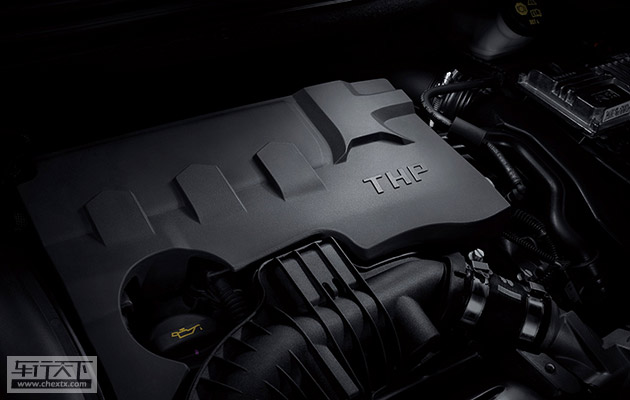东风标致 标致2008

王者归来 标致车队携2008DKR问鼎达喀尔拉力赛

2016年01月25日 13:35 来源：www.chextx.com 超过：78874次关注

1月16日，2016年的汽车赛事开年巨作“达喀尔拉力赛”顺利完赛，由彼得汉塞尔和科特雷驾驶2008DKR经过艰苦奋战，最终摘得冠军荣耀，标致车队完成“王者归来”并一举夺冠的壮举。

标致车队的表现几乎是压倒性的，取得如此优异表现与各项性能大幅提升的2008DKR赛车息息相关。这款富有激情、活力的“战车”凭借其与生俱来的动感基因和出色的SUV属性，征服了被称为“勇敢者的游戏”、“世界上最艰苦”的拉力赛。

携手倾力打造的2008DKR战车参赛使得标致车队如虎添翼，而与2008DKR有着相同的基因，作为“城市SUV领航者”的东风标致2008在场外同样有着极高的人气。为了冠军出发，最多只能获得冠军，对极致探险的热爱才是尽享自由的终极驱动力。复杂艰难的地形，极端无常的天气，不可预知的险境，召唤着永不畏惧的2008DKR。作为出征顶级赛事的战车，2008DKR在地球上最恶劣的行车环境中，迎接性能的极限挑战。与2008DKR有着相同基因的“城市SUV领航者”的东风标致2008在都市丛林中同样驰骋自如。

2008延续了法系车优良的底盘调校，车身悬挂扎实且韧性十足。得益于“T+STT”高效动力，2008在面对一些复杂的路段时，反应更加灵活，它独有的Twin scroll双涡道涡轮增压及高压缸内直喷技术等两项前沿科技，使得2008的爆发力惊人，消费者可以尽情享受SUV所带来的激情动力。

此外，配合Stop-Start发动机智能启停系统，2008实现了低油耗与强动力的完美平衡。其采用了目前最为成熟的“微混”技术，在全面释放高效动力的同时，带来最高可达15%的节油性。据国家工信部实测，2008 1.6THP车型的百公里综合油耗仅为6L。

为了适应赛事需求，作为此次达喀尔拉力赛参赛车辆，2008DKR在车型外观与内饰方面均进行了专业的改装。而东风标致2008则更实实在在符合大众审美，在融合了东风标致品牌全新设计理念以及欧系高性能基因的同时，也恰到好处地融入了各种高科技元素，充分满足了年轻人对时尚、潮流、个性化的追求，无论是独特的“全景天幕玻璃顶”或是“星际宇航驾驶空间”都为普通用户带来了最舒适的驾乘体验。2008搭载的“全景天幕玻璃顶”长达1290mm、宽达1013mm，形成了几乎覆盖整个车顶的1.3平方米的超大面积，并采用无分段结构设计，抬头仰望的瞬间，全景天窗将以360度无死角的超大视野，使都市大玩家们看得尽兴，玩得开心。

“星际宇航驾驶空间”中的手刹挡把形似飞机推进器，这项设计与定速巡航一起操作，会带来驾驶太空飞船的别样感觉，仪表盘的灯光也与飞机类似，科技感极强。7寸超大彩色触摸屏功能强大，不仅有蓝牙电话、显示即时车辆信息等功能，还集娱乐、3D智能导航、实景倒车影像于一身，反应灵敏，功能强大，更操作便捷。

作为东风标致品牌的良心之作，东风标致2008集“低油耗、大视野、强动力”于一身，自上市以来，东风标致2008便跻身都市SUV市场最受关注的车型之一，淋漓地展现了领先同级的产品实力。伴随着2008 DKR战车在达喀尔拉力赛上的激情演出，东风标致2008也必将在新的一年为消费者带来更多惊喜！

0-500 字已有评论 0条 查看评论>>

标致2008猜你喜欢

﻿
• 快速找车
• 选择品牌
• 选择品牌
• A  奥迪
• A  阿斯顿·马丁
• A  阿尔法·罗密欧
• B  宝沃
• B  布加迪
• B  巴博斯
• B  保时捷
• B  宾利
• B  奔驰
• B  宝马
• B  本田
• B  别克
• B  标致
• B  比亚迪
• B  宝骏
• B  北汽制造
• B  北汽新能源
• B  北汽幻速
• B  北汽威旺
• B  北京汽车
• B  奔腾
• B  北汽绅宝
• C  长安
• C  长安商用
• C  长城
• C  昌河
• D  大众
• D  道奇
• D  DS
• D  东南
• D  东风风神
• D  东风风行
• D  东风小康
• D  东风风度
• D  东风
• F  福特
• F  丰田
• F  菲亚特
• F  法拉利
• F  福田
• F  福迪
• F  福汽启腾
• G  观致
• G  广汽传祺
• G  广汽吉奥
• G  GMC
• H  红旗
• H  汉腾汽车
• H  哈弗
• H  哈飞
• H  海格
• H  海马
• H  华颂
• H  黄海
• H  华泰
• H  恒天
• J  吉利汽车
• J  捷豹
• J  Jeep
• J  江淮
• J  江铃
• J  金杯
• J  九龙
• J  金旅
• K  凯翼
• K  凯迪拉克
• K  克莱斯勒
• K  科尼塞克
• K  卡威
• K  开瑞
• L  路虎
• L  林肯
• L  劳斯莱斯
• L  兰博基尼
• L  雷克萨斯
• L  铃木
• L  雷诺
• L  理念
• L  力帆
• L  莲花汽车
• L  猎豹
• L  路特斯
• L  陆风
• M  马自达
• M  MG
• M  MINI
• M  玛莎拉蒂
• M  摩根
• M  迈凯轮
• N  纳智捷
• O  欧宝
• O  讴歌
• O  欧朗
• Q  奇瑞
• Q  起亚
• Q  启辰
• R  日产
• R  荣威
• R  瑞麒
• S  三菱
• S  斯威汽车
• S  萨博
• S  smart
• S  斯柯达
• S  斯巴鲁
• S  思铭
• S  双龙
• S  上汽大通
• S  双环
• T  特斯拉
• T  腾势
• W  沃尔沃
• W  五菱汽车
• W  五十铃
• W  威兹曼
• W  威麟
• X  现代
• X  雪佛兰
• X  雪铁龙
• X  西雅特
• Y  一汽
• Y  英菲尼迪
• Y  英致
• Y  依维柯
• Y  野马汽车
• Y  永源
• Z  众泰
• Z  中华
• Z  中兴
• Z  知豆
• 选择车系
• 选择车系
• 车型对比
• 选择品牌
• 选择品牌
• A  奥迪
• A  阿斯顿·马丁
• A  阿尔法·罗密欧
• B  宝沃
• B  布加迪
• B  巴博斯
• B  保时捷
• B  宾利
• B  奔驰
• B  宝马
• B  本田
• B  别克
• B  标致
• B  比亚迪
• B  宝骏
• B  北汽制造
• B  北汽新能源
• B  北汽幻速
• B  北汽威旺
• B  北京汽车
• B  奔腾
• B  北汽绅宝
• C  长安
• C  长安商用
• C  长城
• C  昌河
• D  大众
• D  道奇
• D  DS
• D  东南
• D  东风风神
• D  东风风行
• D  东风小康
• D  东风风度
• D  东风
• F  福特
• F  丰田
• F  菲亚特
• F  法拉利
• F  福田
• F  福迪
• F  福汽启腾
• G  观致
• G  广汽传祺
• G  广汽吉奥
• G  GMC
• H  红旗
• H  汉腾汽车
• H  哈弗
• H  哈飞
• H  海格
• H  海马
• H  华颂
• H  黄海
• H  华泰
• H  恒天
• J  吉利汽车
• J  捷豹
• J  Jeep
• J  江淮
• J  江铃
• J  金杯
• J  九龙
• J  金旅
• K  凯翼
• K  凯迪拉克
• K  克莱斯勒
• K  科尼塞克
• K  卡威
• K  开瑞
• L  路虎
• L  林肯
• L  劳斯莱斯
• L  兰博基尼
• L  雷克萨斯
• L  铃木
• L  雷诺
• L  理念
• L  力帆
• L  莲花汽车
• L  猎豹
• L  路特斯
• L  陆风
• M  马自达
• M  MG
• M  MINI
• M  玛莎拉蒂
• M  摩根
• M  迈凯轮
• N  纳智捷
• O  欧宝
• O  讴歌
• O  欧朗
• Q  奇瑞
• Q  起亚
• Q  启辰
• R  日产
• R  荣威
• R  瑞麒
• S  三菱
• S  斯威汽车
• S  萨博
• S  smart
• S  斯柯达
• S  斯巴鲁
• S  思铭
• S  双龙
• S  上汽大通
• S  双环
• T  特斯拉
• T  腾势
• W  沃尔沃
• W  五菱汽车
• W  五十铃
• W  威兹曼
• W  威麟
• X  现代
• X  雪佛兰
• X  雪铁龙
• X  西雅特
• Y  一汽
• Y  英菲尼迪
• Y  英致
• Y  依维柯
• Y  野马汽车
• Y  永源
• Z  众泰
• Z  中华
• Z  中兴
• Z  知豆
• 选择车系
• 选择车系
• 选择车型
• 选择车型
• 意见反馈